# Motion and Time Types of Motions CBSE

Speed

• The distance covered by an object in unit time is defined as speed.
• What do we mean when we say a car is going at a speed of 80 km/hr?
• It means the car having such will cover a distance of 80 km in 1 hour.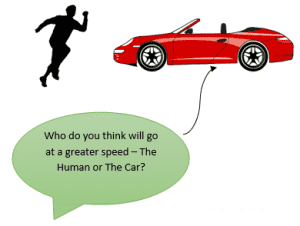Speed can also be defined as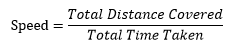## Uniform Motion and Non Uniform Motion## Measurement of time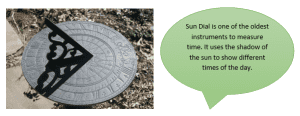• Imagine the time before the invention of clocks. Ever wondered how our ancestors used to determine the time? While travelling from one place to another, how would have they calculated the time?
• Our ancestors used the repetitive nature of natural occurrences to predict time like sunrise and sunset, new moon to full moon.
• Can we create anything that has a repetitive/periodic nature and use it to measure time? A sun dial, a simple pendulum can be examples of such instruments.

## A simple pendulum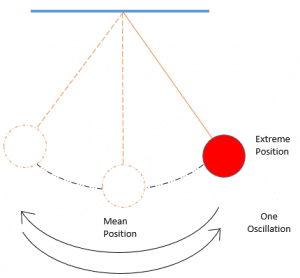• Time taken by the pendulum to complete one oscillation is known as time period.
• Suppose a person is running on a track. We can calculate the time taken by the man to run on the track in terms of number of oscillations of the pendulum.
• For e.g.a person ran 400 Metres in 5 oscillations of simple pendulum.

## Units of time and speed

• The basic unit of time is second and is denoted by s.
• The basic unit of Distance is metre and is denoted by m.
• As , basic unit of speed is metre/second or m/s.
• 1000 Metre = 1 Kilometer (Km)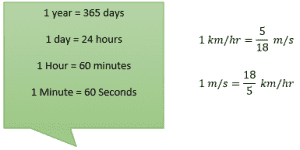## Measuring Speed and Distance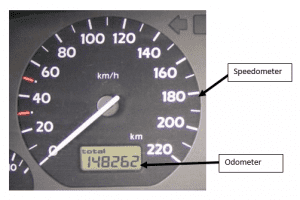• Speedometer – Measures Speed
• Odometer – Measures Distance

Distance Time graph

Illustration

Consider the motion of a car going at a constant speed on a straight road. Given below is the distance covered with respect to time. Draw the distance time graph for the data given below. Also calculate the distance covered at 10:30 PM.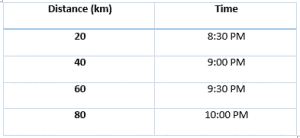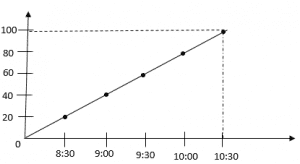From the graph we can say that the car will travel 100 km at 10:30 PM.

From the above discussion, students would be able to understand the link between motion and time. But to deepen the knowledge, it is essential to practice what you have learned by attempting exercises like the one mentioned here- NCERT solutions for motion and time.